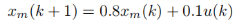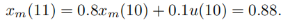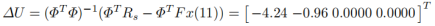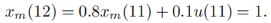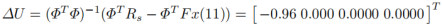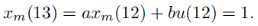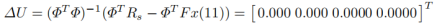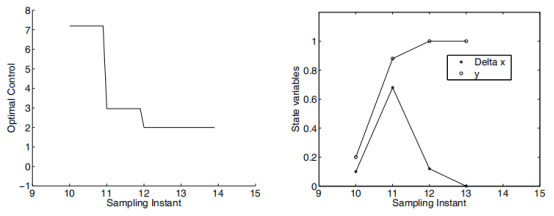### 4.1 闭环控制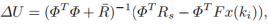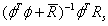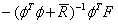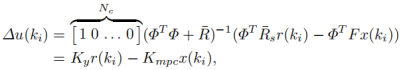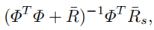K m p c  是下式的第一行：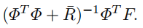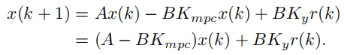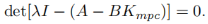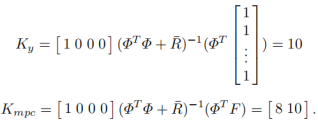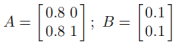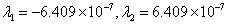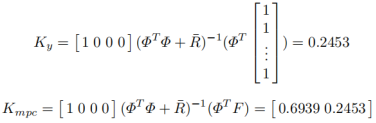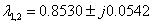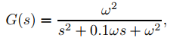omega=10;
numc=omega^2;
denc=[1 0.1*omega omega^2];
[Ac,Bc,Cc,Dc]=tf2ss(numc,denc);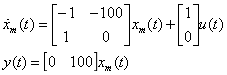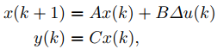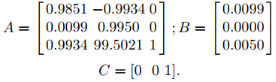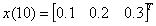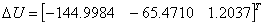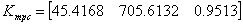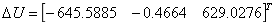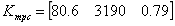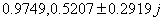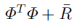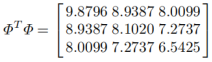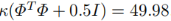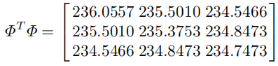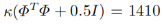### 4.2 Matlab实现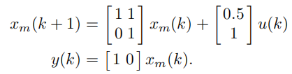Ap=[1 1;0 1];
Bp=[0.5;1];
Cp=[1 0];
Dp=0;
Np=20;
Nc=4;


[Phi_Phi,Phi_F,Phi_R,A_e, B_e,C_e] = mpcgain(Ap,Bp,Cp,Nc,Np);
[n,n_in]=size(B_e);
xm=[0;0];
Xf=zeros(n,1);
N_sim=100;
r=ones(N_sim,1);
u=0; % u(k-1) =0
y=0;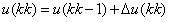for kk=1:N_sim;
DeltaU=inv(Phi_Phi+0.1*eye(Nc,Nc))*(Phi_R*r(kk)-Phi_F*Xf);
deltau=DeltaU(1,1);
u=u+deltau;
u1(kk)=u;
y1(kk)=y;

xm_old=xm;
xm=Ap*xm+Bp*u;
y=Cp*xm;
Xf=[xm-xm_old;y];
end

k=0:(N_sim-1);
figure
subplot(211)
plot(k,y1)
xlabel('Sampling Instant')
legend('Output')
subplot(212)
plot(k,u1)
xlabel('Sampling Instant')
legend('Control')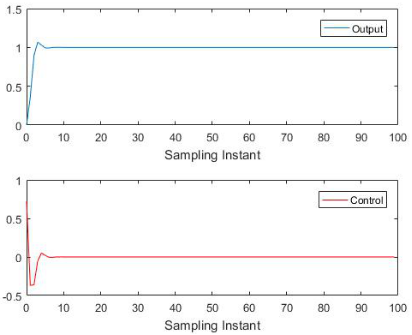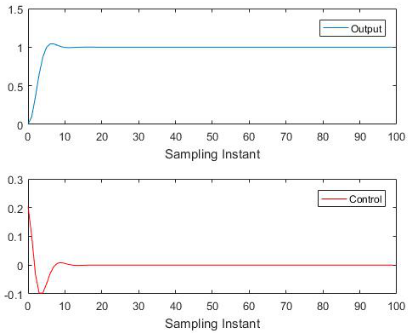Liuping Wang. Model Predictive Control System Design and Implementation Using MATLAB.